NCERT Solutions: Surface Areas & Volumes (Exercise 12.3)

# Surface Areas & Volumes (Exercise 12.3) NCERT Solutions - Mathematics (Maths) Class 10

[Take π = 22/7, (Unless stated otherwise)]
Q 1. A metallic sphere of radius 4.2 cm is melted and recast into the shape of a cylinder of radius 6 cm. Find the height of the cylinder.
Sol. Radius of the sphere (r1) = 4.2 cm
∴ Volume of the sphere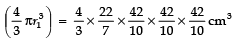Radius of the cylinder (r2) = 6 cm
Let ‘h’ be the height of the cylinder
∴ Volume of the cylinder =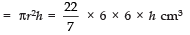Since, Volume of the metallic sphere = Volume of the cylinder.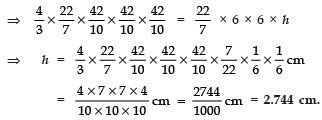Q 2. Metallic spheres of radii 6 cm, 8 cm and 10 cm, respectively, are melted to form a single solid sphere. Find the radius of the resulting sphere.
Sol. Radii of the given spheres are:
r1 = 6 cm
r2 = 8 cm
r3 = 10 cm
⇒ Volume of the given spheres are: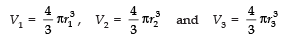∴ Total volume of the given spheres
= V1 + V2 + V3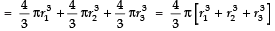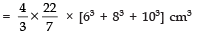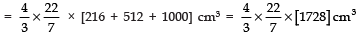Let the radius of the new big sphere be R.
∴ Volume of the new sphere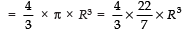Since the two volumes must be equal.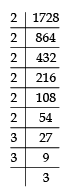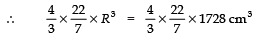⇒ R3 = 1728
⇒ R3 =23 × 23 × 33
⇒ R3 = (2 × 2 × 3)3
⇒ R = 2 × 2 × 3
⇒ R = 12 cm
Thus, the required radius of the resulting sphere = 12 cm.

Q 3. A 20 m deep well with a diameter 7 m is dug and the earth from digging is evenly spread out to form a platform 22 m by 14 m. Find the height of the platform.
Sol. Given, diameter of the well = 7m
and depth of the well = 20 m
Volume of the earth taken out from the well = πr2h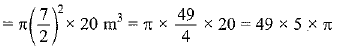Earth is evenly spread on a platform 22 m by 14 m
Let the height of platform be h
∴ Volume of platform = 22 x 14 x h
According to the question,
Volume of the platform = Volume of the earth taken out from the well
⇒ 22 x 14 x h = π x 49 x 5
⇒ 22 x 14 x h = 22/7 x 49 x 5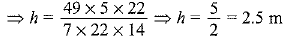Q 4. A well of diameter 3 m is dug 14 m deep. The earth taken out of it has been spread evenly all around it in the shape of a circular ring of width 4 m to form an embankment. Find the height of the embankment.
Sol. Diameter of cylindrical well (d) = 3 m
⇒ Radius of the cylindrical well (r) = 3/2 m = 1.5m
Depth of the well (h) = 14 m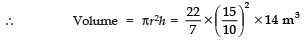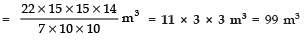Let the height of the embankment = ‘H’ meter
Internal radius of the embankment (r) = 1.5 m.
External radius of the embankment R = (4 + 1.5) m = 5.5 m.
∴ Volume of the embankment
= πR2H − πr2H = πH [R2 − r2] = πH (R + r) (R − r)
= 22/7 × H (5.5 + 1.5) (5.5 − 1.5) = 22/7 × H × 7 × 4 m3
Since, Volume of the embankment = Volume of the cylindrical well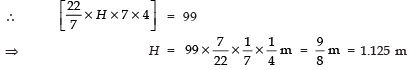Thus, the required height of the embankment = 1.125 m.

Q 5. A container shaped like a right circular cylinder having a diameter 12 cm and height 15 cm is full of ice cream. The ice cream is to be filled into cones of height 12 cm and diameter 6 cm, having a hemispherical shape on the top. Find the number of such cones which can be filled with ice cream.
Sol. For the circular cylinder:
Diameter = 12 cm ⇒ Radius = 12/2 =  6 cm
Height (h) = 15 cm
∴ Volume = πr2h
⇒ Volume of total ice cream = 22/7 × 6 × 6 × 15 cm3
For conical + hemispherical ice-cream cone:
Diameter = 6 cm ⇒ radius (R) = 3 cm
Height of ice cream cone (H) = 12 cm
Volume = (Volume of the conical part) + (Volume of the hemispherical part)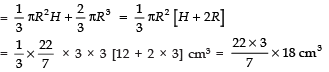Let number of ice-cream cone required to fill the total ice cream = n.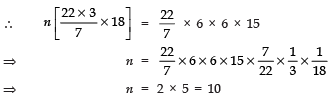Thus, the required number of cones is 10.

Q 6. How many silver coins, 1.75 cm in diameter and of thickness 2 mm, must be melted to form a cuboid of dimensions 5.5 cm × 10 cm × 3.5 cm?
Sol. For a circular coin:
Diameter = 1.75 cm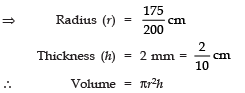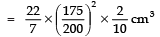|∵ A coin is like a cylinder
For a cuboid:
Length (l) = 10 cm, Breadth (b) = 5.5 cm
and Height (h) = 3.5 cm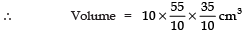Number of coins
Let the number of coins need to melt be ‘n’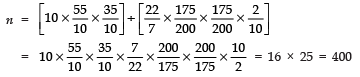Thus, the required number of coins = 400.

Q 7. A cylindrical bucket, 32 cm high and with radius of base 18 cm, is filled with sand. This bucket is emptied on the ground and a conical heap of sand is formed. If the height of the conical heap is 24 cm, find the radius and slant height of the heap.
Sol. For the cylindrical bucket:
Height (h) = 32 cm
Volume = πr2h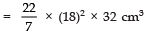⇒ Volume of the sand =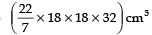For the conical heap:
Height (H) = 24 cm
Let radius of the base be (R).
∴ Volume of conical heap =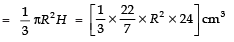Radius of the conical heap of sand:
∵ Volume of the conical heap of sand = Volume of the sand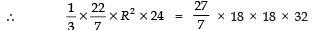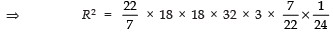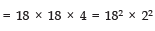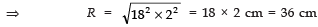Slant Height
Let ‘l’ be the slant height of the conical heap of the sand.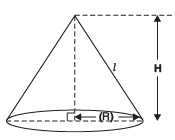∴ l2 = R2 + H2
⇒ l2 =242 + 362
⇒ l2 = (12 × 2)2 + (12 × 3)2
⇒ l2 =122 [22 + 32]
⇒ l2 =122 × 13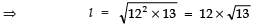Thus, the required height = 36 cm and slant height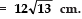Q 8. Water in a canal, 6 m wide and 1.5 m deep, is flowing with a speed of 10 km/h. How much area will it irrigate in 30 minutes, if 8 cm of standing water is needed?
Sol. Width of the canal = 6 m
Depth of the canal = 1.5 m
Length of the water column in 1 hr = 10 km
∴ Length of the water column in 30 minutes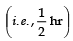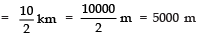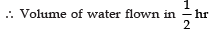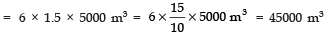Since the above amount (volume) of water is spread in the form of a cuboid of height as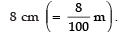Let the area of the cuboid = a
∴ Volume of the cuboid=Area × Height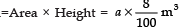Thus,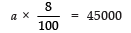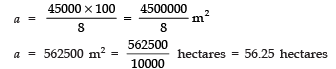Thus, the required area = 56.25 hectares.

Q 9. A farmer connects a pipe of internal diameter 20 cm from a canal into a cylindrical tank in her field, which is 10 m in diameter and 2 m deep. If water flows through the pipe at the rate of 3 km/h, in how much time will the tank be filled?
Sol.  Given, diameter of pipe = 20 cm
Water flowing from the pipe at rate = 3 km/h
Let it filled the tank in t hours.
Volume of water flowing in t hours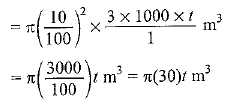Volume of the water in cylindrical tank = πr2h = π(5)x 2 m3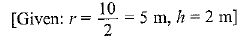According to the question,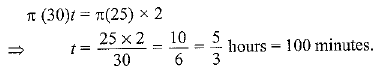The document Surface Areas & Volumes (Exercise 12.3) NCERT Solutions | Mathematics (Maths) Class 10 is a part of the Class 10 Course Mathematics (Maths) Class 10.
All you need of Class 10 at this link: Class 10

## Mathematics (Maths) Class 10

115 videos|478 docs|129 tests

## FAQs on Surface Areas & Volumes (Exercise 12.3) NCERT Solutions - Mathematics (Maths) Class 10

 1. What are the formulas for finding the surface area and volume of a cube?Ans. The surface area of a cube is given by the formula 6a^2, where 'a' is the length of the side. The volume of a cube is given by the formula a^3, where 'a' is the length of the side.
 2. How can I find the surface area of a cylinder?Ans. The surface area of a cylinder can be found by adding the areas of its two circular bases and the area of its curved surface. The formula for the surface area of a cylinder is 2πr^2 + 2πrh, where 'r' is the radius of the base and 'h' is the height of the cylinder.
 3. How do I calculate the volume of a cone?Ans. The volume of a cone can be calculated using the formula (1/3)πr^2h, where 'r' is the radius of the base and 'h' is the height of the cone.
 4. What is the surface area of a sphere?Ans. The surface area of a sphere can be calculated using the formula 4πr^2, where 'r' is the radius of the sphere.
 5. How can I find the volume of a cuboid?Ans. The volume of a cuboid can be found by multiplying its length, width, and height. The formula for the volume of a cuboid is lwh, where 'l' is the length, 'w' is the width, and 'h' is the height of the cuboid.

## Mathematics (Maths) Class 10

115 videos|478 docs|129 testsExplore Courses for Class 10 examSignup to see your scores go up within 7 days! Learn & Practice with 1000+ FREE Notes, Videos & Tests.
10M+ students study on EduRev
Track your progress, build streaks, highlight & save important lessons and more!
Related Searches

,

,

,

,

,

,

,

,

,

,

,

,

,

,

,

,

,

,

,

,

,

;